##### Microeconomics For Dummies - UK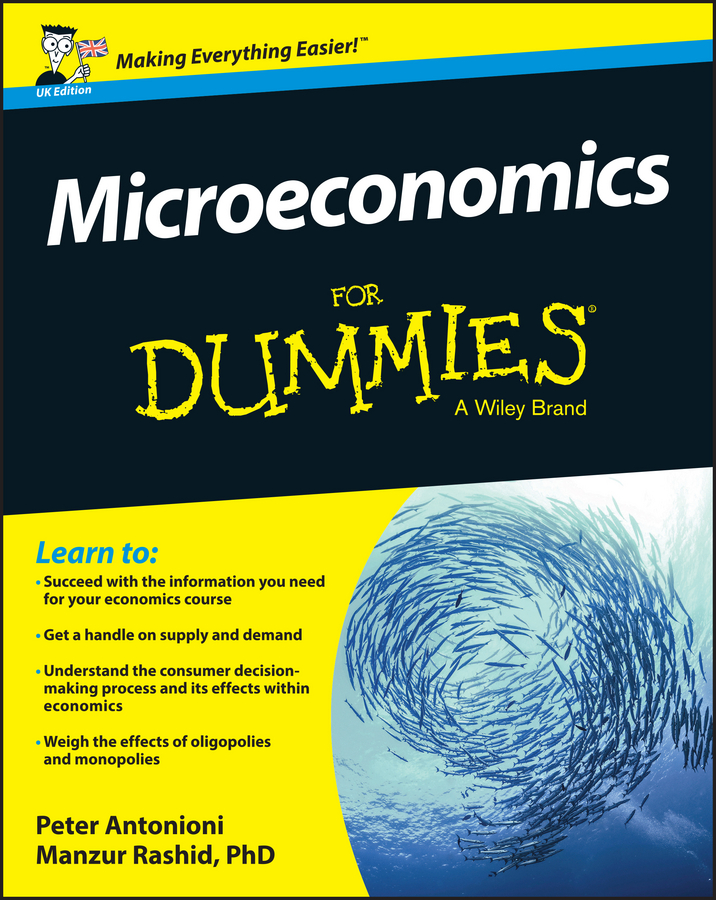Monopolists, as is the case for many other firms, often produce their product in more than one factory. In order to maximize profits, the monopolist must determine how to allocate production among these factories.

In determining this allocation, the monopolist’s goal is to produce additional units at the lowest cost. Therefore, the monopolist produces additional units of the product in the factory that has the lowest marginal cost. As a result, the monopolist minimizes the total cost of producing the total amount of the product.

If the marginal cost of producing an additional unit of output in one factory is higher than the marginal cost of producing the additional unit of output in a second factory, you’re not minimizing total production costs. Your total costs are lower if you switch production from the factory with the higher marginal cost to the factory with the lower marginal cost.

Cost minimization requires that the marginal cost of the last unit produced in each factory is equal for all factories. The illustration shows cost minimization for a monopoly with two factories. The marginal cost curve in the far right diagram labeled ”MC is the horizontal summation of the marginal cost curves for each factory.

The illustration shows two factories, A and B. Thus, marginal cost equals MC* is associated with the output level qA1 in factory A and the output level qB2 in factory B. For the monopoly, it produces the output level q* at a marginal cost MC*. The output q* simply is the horizontal summation of the quantities each factory produces given MC*, or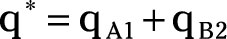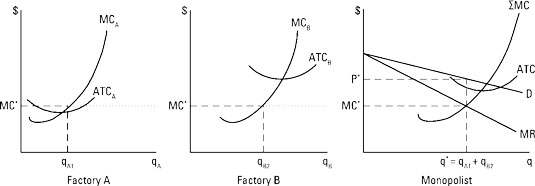Note in the illustration the monopolist wants to produce the output level q* and charge the price P* in order to maximize profits, because marginal revenue, MR, equals marginal cost, MC, in the far right diagram.

In order to minimize the cost of producing q*, the monopolist must produce the output level in each factory that corresponds to MC*. Thus, q1 units are produced in factory A and q2 units are produced in factory B.

## How to calculate the best allocation

Calculus precisely determines the amount of output to produce in each factory — something that is difficult to determine graphically. Profit-maximizing production with multiple factories requires the satisfaction of the following equation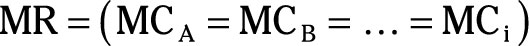In other words, profit maximization requires that the monopolist’s overall marginal revenue, MR, equals the marginal cost of production at each of its factories — factory A, factory B, and out to factory I — however many factories there are.

Assume the following equations describe a monopolist’s demand, total revenue, and marginal revenue curves: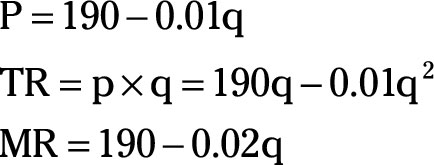The firm has two factories. Factory A’s total cost and marginal cost equations are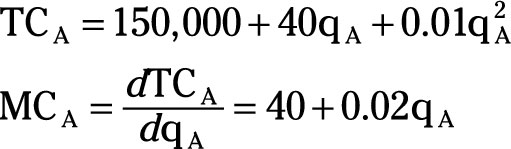Factory B’s total cost and marginal cost equations are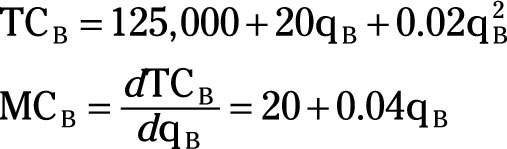As indicated, profit-maximization requires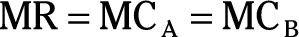By solving this set of equations simultaneously, the monopolist’s profit-maximizing quantity of output is determined, as well as the quantity of output that’s produced in each factory.

1. Set MCA = MCB and solve for qA as a function of qB.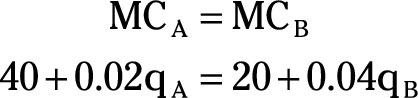2. Set MR = MCB.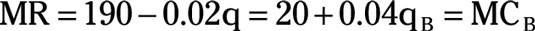3. Substitute qA + qB for q.

Because the total quantity of output the monopolist sells, q, is produced in some combination from factories A and B, the quantities produced in each factory added together must equal the quantity sold by the monopolist.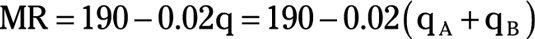4. Substitute qA = –1,000 + 2qB from Step 1 for qA in Step 3’s equation.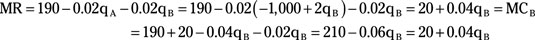5. Solve for qB.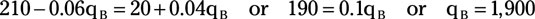6. Using the equation from Step 1, solve for qA.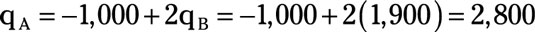7. Solve for q, the total quantity of output the monopolist sells.8. Solve for P, the price the monopolist establishes.

P is determined by using the demand equation at the beginning of the example.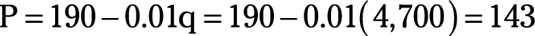9. The monopolist’s total profit is determined by subtracting the total cost of producing the given output in each factory from total revenue.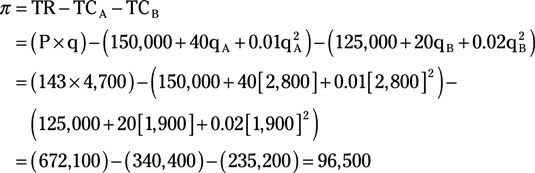So, the monopolist’s total profit is \$96,500. Knowing how to minimize cost when producing in two or more facilities is even better than collecting \$200 when you pass “Go” in the board game Monopoly.